# Line Voltage to Phase Voltage Line Current to Phase Current Relationship:

## What is Line Voltage:

In three phase power system, The potential difference between the two phases is called line voltage (typically phase to phase). It is denoted by VL-L . The voltage between R to Y or Y to B or B to R. In power system, system voltage means line to line voltage. Refer the Diagram,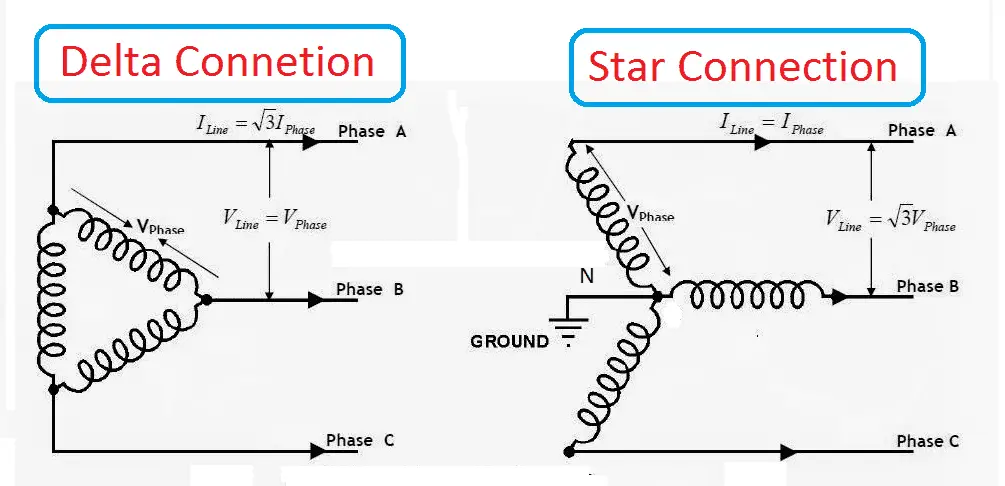Example: our domestic power supply is three phase, 440 Volts. Here 440 volts means the phase to phase voltage is 440.

Note: If they mention in single phase 230 volts, then it means the potential difference the phase to neutral is 230 volts.

In star connection: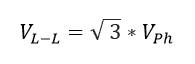Line voltage = 1.732 times of phase voltage.

In delta connection: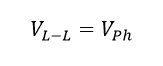Line voltage = Phase voltage.

## What is line current:

The measure of current in one phase before the star or delta arrangement of the component is called line current (typically input current in motor or output current in alternator). In three phase balanced system, it may be R phase current or Y phase current or B phase current.

It is denoted by IL ampere.

In star connection: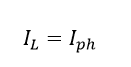Line current= phase current. (we get it from applying Kirchhoff’s current rule.)

In delta connection: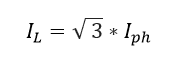Line current= phase current. (we get it from applying Kirchhoff’s voltage rule.)

## What is phase voltage:

In three phase system, the potential difference between one phase to natural point is called phase voltage. It is denoted by Vph volts

Star Connection: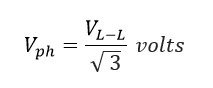Phase voltage = Line voltage divide by 1.732

In Delta Connection:

## Phase current:

Phase current is the measure of current inside of star connection or delta connection of the three phase system. It is denoted by Iph.

In star connection: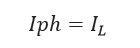Phase current = Line current

In delta connection: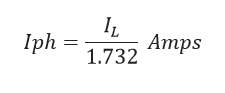Note: Value of3 = 1.732.

Do You Want any Article related to electrical Topic, Comment Us with the Topic Name and We provide it for you..